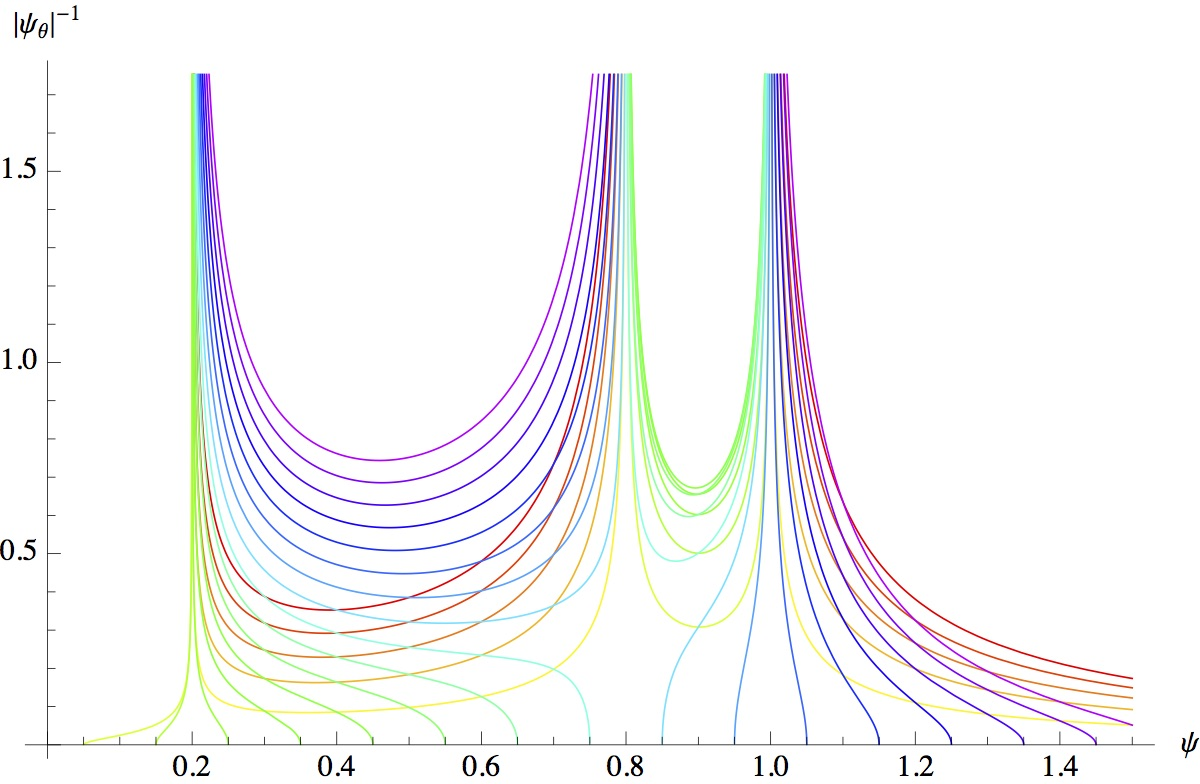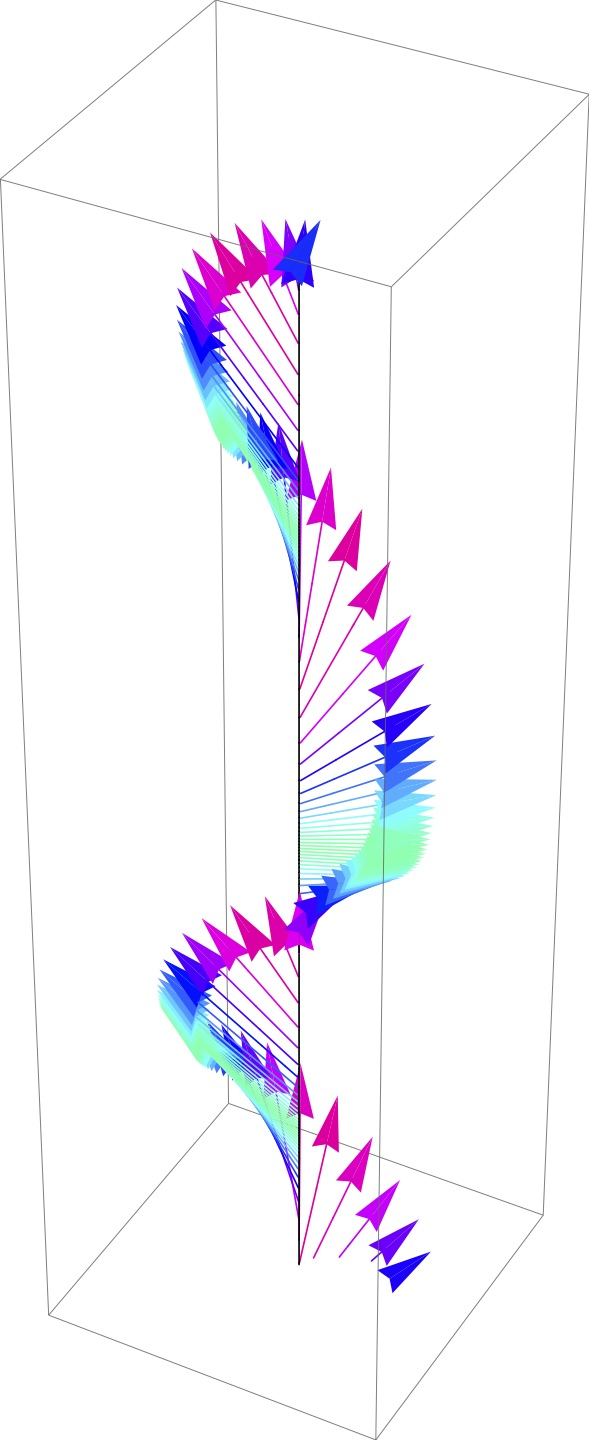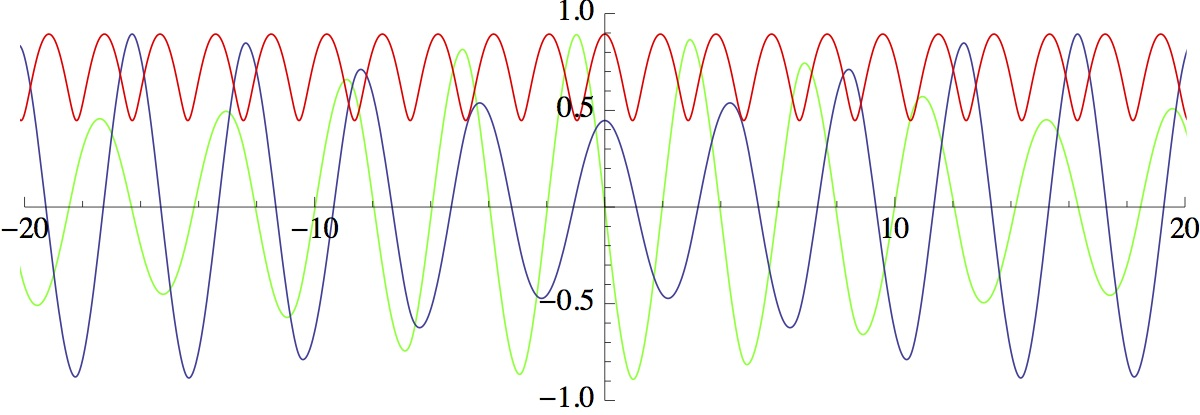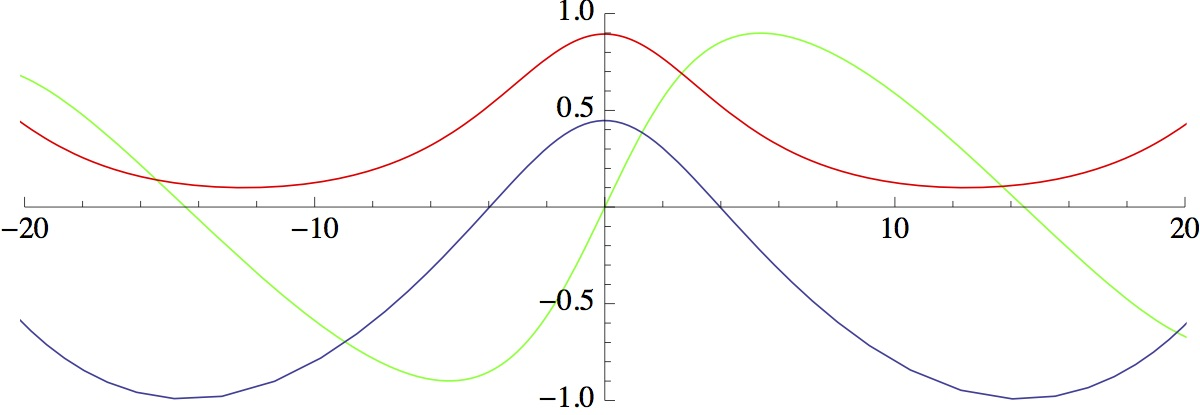MMNLP
Mathematical Methods of NonLinear Physics

## Abstract

We deal with the construction and investigation of completely integrable nonlinear equations, or so close to being integrable to allow for investigation by analytic and/or perturbative techniques, which are usually good models of classical or quantum systems of applicative relevance (models in plasmas, nuclear physics, optics and condensed matter, nonlinear phenomena in gravitational field theory, spin chains, etc..). These integrable, or quasi-integrable model equations may be defined on continuous or discrete sets, as systems of partial, ordinary differential, or difference equations. Within this general framework, our main research interests cover the following topics.

• Development of methods of solution for initial-boundary value problems and direct approaches to construct exact solutions;
• analysis of the singular behavior of solutions of integrable equations of hydrodynamic type, connection with the general singularity theory, and the problem of dispersive regularization and shock formation;
• classification and construction of classes of integrable equations and systems, and its relation with classes of deformations of algebraic curves and varieties;
• construction and investigation of classical and quantum integrable dynamical systems, and their integrable time-discretizations;
• integrable and linearizable nonlinear difference and partial difference equations, and the discretization of differential systems with symmetries;
• application of the theory of integrable equations to the study of various nonlinear models in classical and quantum field theory.
Most suitable mathematical methods are connected to spectral analysis of operators in finite or infinite dimensional spaces (f.i., Lax pairs, Inverse Spectral Transforms, linear and nonlinear Riemann-Hilbert problems), and algebraic techniques (f.i., Lie-point and generalized symmetries, Hamiltonian structures).Inserire didascaliaInserire didascaliaInserire didascaliaInserire didascalia